# 2.4 – A Simple PDF Example

Previous: 2.3 – The Probability Density Function

Next: 2.5 – Some Common Continuous Distributions

## Question

Let f(x) = k(3x2 + 1).

1. Find the value of k that makes the given function a PDF on the interval 0 ≤ x ≤ 2.
2. Let X be a continuous random variable whose PDF is f(x). Compute the probability that X is between 1 and 2.
3. Find the distribution function of X.
4. Find the probability that X is exactly equal to 1.

## Solution

### Part 1)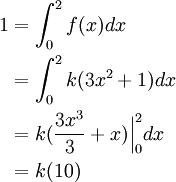Therefore, k = 1/10.

Notice that f(x) ≥ 0 for all x. Also notice that we can rewrite this PDF in the obvious way so that it is defined for all real numbers: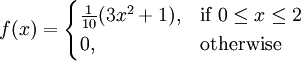### Part 2)

Using our value for k from Part 1: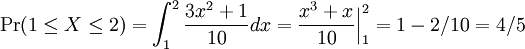Therefore, Pr(1 ≤ X ≤ 2) is 4/5.

### Part 3)

Using the Fundamental Theorem of Calculus, the CDF of X at x in [0,2] is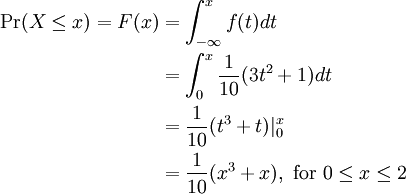We can also easily verify that F(x) = 0 for all x < 0 and that F(x) = 1 for all x > 2.

### Part 4)

Since X is a continuous random variable, we immediately know that the probability that it equals any one particular value must be zero. More directly, we compute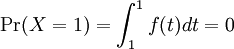Previous: 2.3 – The Probability Density Function

Next: 2.5 – Some Common Continuous Distributions